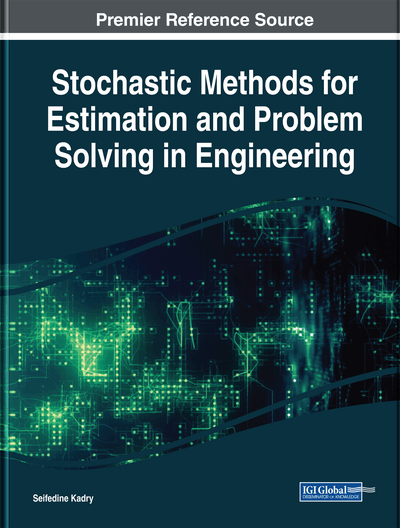# Stochastic Methods Applied to Structural Mechanics: Reliability and Optimization Methods

Siham Ouhimmou (University Hassan II, Morocco)
DOI: 10.4018/978-1-5225-5045-7.ch009

## Abstract

Uncertainty modelling with random variables motivates the adoption of advanced PTM for reliability analysis to solve problems of mechanical systems. Probabilistic transformation method (PTM) is readily applicable when the function between the input and the output of the system is explicit. When these functions are implicit, a technique is proposed that combines finite element analysis (FEA) and probabilistic transformation method (PTM) that is based on the numerical simulations of the finite element analysis (FEA) and the probabilistic transformation method (PTM) using an interface between finite element software and Matlab. Structure problems are treated with the proposed technique, and the obtained results are compared to those obtained by the reference Monte Carlo method. A second aim of this work is to develop an algorithm of global optimization using the local method SQP. The proposed approach MSQP is tested on test functions comparing with other methods, and it is used to resolve a structural problem under reliability constraints.
Chapter Preview
Top

## Introduction

The lack of penetration of rational uncertainty analysis methods in engineering practice is hardly due to insufficient theoretical foundations or the scarcity of efficient algorithms. Indeed, uncertainty and reliability analysis in structural engineering have been vibrant topics of research for several decades (Mohamed &Lemaire, 1995; Der Kiureghian & Ke, 1988; Lemaire, Mohamed, & Flores-Macias, 1997). While a large portion of the associated efforts have focused on shedding light on the fundamental and theoretical aspects and on the application of the uncertainty analysis methods to strongly simplified, reduced-order models of structures, significant progress has also been made recently in the rational treatment of uncertainties in large FE models of complex structures. The structural designer must verify, within a prescribed safety level, the serviceability and ultimate conditions, commonly expressed by this inequalityWhererepresents the action effect, andis the resistance. The intrinsic random nature of material properties and actions is actually considered by the Eurocodes (Mohamed & Lemaire, 1995; Kadry, 2006), which classify the methods available to deal with this randomness in three levels:

A fundamental problem in structural reliability analysis is the computation of the probability integral,

(1)

Wherein whichis the transpose, is a vector of random variables representing the uncertain parameters of considered structure,is the probability density function of,is the Limit State Function defined such that:is the domain of integration denoted the failure set, andis the probability of failure. The difficulty of computing this integration led to development of various methods of reliability analysis such as Monte Carlo, FORM and SORM (Hasofer & Lind, 1974, Muscolino, Ricciardi, & Impollonia, 2000), and Probabilistic Transformation Method PTM (Lemaire, Mohamed, & Flores-Macias, 1997).

## Complete Chapter List

Search this Book:
Reset